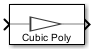# Memoryless Nonlinearity

Apply memoryless nonlinearity to complex baseband signal

• Library:
• Communications Toolbox / RF Impairments

•## Description

The Memoryless Nonlinearity block applies memoryless nonlinear impairments to a complex baseband signal. Use this block to model memoryless nonlinear impairments caused by signal amplification in the radio frequency (RF) transmitter or receiver. For more information, see Memoryless Nonlinear Impairments.

### Note

All values of power assume a nominal impedance of 1 ohm.

## Ports

### Input

expand all

Input RF baseband signal, specified as a numeric scalar or numeric column vector. Values in this input must be complex.

Data Types: `double`
Complex Number Support: Yes

### Output

expand all

Output RF baseband signal, returned as a numeric scalar or numeric column vector. The output is of the same data type as the input.

## Parameters

expand all

Nonlinearity modeling method, specified as `Cubic polynomial`, `Hyperbolic tangent`, `Saleh model`, `Ghorbani model`, `Rapp model`, or `Lookup table`. For more information, see Memoryless Nonlinear Impairments.

Linear gain in decibels, specified as a numeric scalar. This parameter scales the power gain of the output signal.

Tunable: Yes

#### Dependencies

To enable this parameter, set the Method to `Cubic polynomial`, ```Hyperbolic tangent```, or `Rapp model`.

Data Types: `double`

Third-order input intercept point in dBm, specified as a numeric scalar.

Tunable: Yes

#### Dependencies

To enable this parameter, set the Method to `Cubic polynomial` or ```Hyperbolic tangent```.

Data Types: `double`

AM/PM conversion factor in degrees per decibel, specified as a numeric scalar. For more information, see Cubic Polynomial and Hyperbolic Tangent Model Methods.

Tunable: Yes

#### Dependencies

To enable this parameter, set the Method to `Cubic polynomial` or ```Hyperbolic tangent```.

Data Types: `double`

Input power lower limit in dBm, specified as a numeric scalar less than the Upper input power limit for AM/PM conversion (dBm) parameter value. The AM/PM conversion scales linearly for input power values in the range [Lower input power limit for AM/PM conversion (dBm), Upper input power limit for AM/PM conversion (dBm)]. If the input signal power is below the input power lower limit, the phase shift resulting from AM/PM conversion is zero. For more information, see Cubic Polynomial and Hyperbolic Tangent Model Methods.

Tunable: Yes

#### Dependencies

To enable this parameter, set the Method to `Cubic polynomial` or `Hyperbolic tangent`.

Data Types: `double`

Input power upper limit in dBm, specified as a numeric scalar greater than the Lower input power limit for AM/PM conversion (dBm) parameter value. The AM/PM conversion scales linearly for input power values in the range [Lower input power limit for AM/PM conversion (dBm), Upper input power limit for AM/PM conversion (dBm)]. If the input signal power is below the input power lower limit, the phase shift resulting from AM/PM conversion is zero. For more information, see Cubic Polynomial and Hyperbolic Tangent Model Methods.

Tunable: Yes

#### Dependencies

To enable this parameter, set the Method to `Cubic polynomial` or `Hyperbolic tangent`.

Data Types: `double`

Input signal scaling factor in decibels, specified as a numeric scalar. This parameter scales the power gain of the input signal.

Tunable: Yes

#### Dependencies

To enable this parameter, set the Method to `Saleh model` or ```Ghorbani model```.

Data Types: `double`

AM/AM parameters for Saleh model, used to compute the amplitude gain for an input signal, specified as a two-element numeric vector. For more information, see Saleh Model Method.

Tunable: Yes

#### Dependencies

To enable this parameter, set the Method to `Saleh model`.

Data Types: `double`

AM/PM parameters for Saleh model, used to compute the phase change for an input signal, specified as a two-element numeric vector. For more information, see Saleh Model Method.

Tunable: Yes

#### Dependencies

To enable this parameter, set the Method to `Saleh model`.

AM/AM parameters for Ghorbani model, used to compute the amplitude gain for an input signal, specified as a four-element numeric vector. For more information, see Ghorbani Model Method.

Tunable: Yes

#### Dependencies

To enable this parameter, set the Method to `Ghorbani model`.

Data Types: `double`

AM/PM parameters for Ghorbani model, used to compute the phase change for an input signal, specified as a four-element numeric vector. For more information, see Ghorbani Model Method.

Tunable: Yes

#### Dependencies

To enable this parameter, set the Method to `Ghorbani model`.

Data Types: `double`

Output signal scaling factor in decibels, specified as a numeric scalar. This parameter scales the power gain of the output signal.

Tunable: Yes

#### Dependencies

To enable this parameter, set the Method to `Saleh model` or ```Ghorbani model```.

Data Types: `double`

Smoothness factor, specified as a numeric scalar. For more information, see Rapp Model Method.

Tunable: Yes

#### Dependencies

To enable this parameter, set the Method to `Rapp model`.

Data Types: `double`

Output saturation level, specified as a numeric scalar. For more information, see Rapp Model Method.

Tunable: Yes

#### Dependencies

To enable this parameter, set the Method to `Rapp model`.

Data Types: `double`

## Block Characteristics

 Data Types `double` | `single` Multidimensional Signals `no` Variable-Size Signals `no`